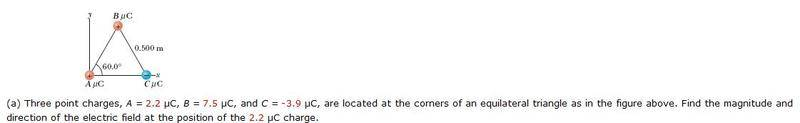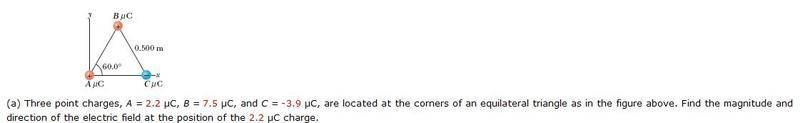# Coulomb's Law problem with three charged points

NotCarlSagan

## Homework StatementF=Keq1q2/r2

## The Attempt at a Solution

Using Coulomb's Law, here's what I've worked out:

FAB=0.593N
FAC=0.309N

Fx=0.309 - 0.593cos60°= 0.0125
Fy=0.593sin60° =0.514

√(0.01252 + 0.5142) = 0.514 N/C

and θ = tan-1(0.514/0.0125) = 88.6°

I'm not sure why webassign is telling me that my answer is wrong. I worked out a problem almost exactly like this with slightly different numbers and got it right...

## Answers and Replies

Nessdude14
You're finding the force on particle A, but the problem asks for the E-field at the position of particle A. Do you know the equation for the E-field of a point charge?

NotCarlSagan
I thought it was the same formula? Shoot. Idk.

I'll review the problem and post here again if I have trouble. I guess I was just looking for the wrong thing. Thanks for pointing that out.

Nessdude14
I thought it was the same formula? Shoot. Idk.
I'll review the problem and post here again if I have trouble. I guess I was just looking for the wrong thing. Thanks for pointing that out.

It's a similar formula, but the E-field has units of N/C (while the equation you used gives force in N).

Hope that helps. If you have more trouble with your problem don't hesitate to ask.

NotCarlSagan
So would the E-field simply be:

E=ke(2.2x10-6)/(0.52) = 7.91 x 104 N/C?

Staff Emeritus
Homework Helper
Gold Member

## Homework StatementF=Keq1q2/r2

## The Attempt at a Solution

Using Coulomb's Law, here's what I've worked out:

FAB=0.593N
FAC=0.309N

Fx=0.309 - 0.593cos60°= 0.0125
Fy=0.593sin60° =0.514

√(0.01252 + 0.5142) = 0.514 N/C

and θ = tan-1(0.514/0.0125) = 88.6°

I'm not sure why webassign is telling me that my answer is wrong. I worked out a problem almost exactly like this with slightly different numbers and got it right...
Webassign is right to tell you it's wrong.

However, Webassign (or whoever wrote the problem) should have asked for the electric field at the position of the 2.2μC due to the two other charges.

Back to your issue ...

What is the direction of the electric field due to charge B?

What is the direction of the electric field due to charge C?

NotCarlSagan
Ok, I gave it a try but I think I'm still wrong.

EA = 7.91 x 104 N/C
EB = 2.70 x 105 N/C
EC = 1.40 x 105 N/C

EAX = (2.70 x 105) + (7.91 x 104)cos 60 = 3.10 x 105

EAY = (2.70 x 105) + (7.91 x 104)sin 60 = 3.39 x 105

ECX = (2.70 x 105) - (1.40 x 105)cos 60 = 2.00 x 105

ECY = (2.70 x 105) - (1.40 x 105)sin 60 = 1.49 x 105

Ex = EAX - ECX = 3.10 x 105 - 2.00 x 105 = 1.10 x 105

EY = EAY - ECY = 1.90 x 105

So, √[(1.10 x 105)2 + (1.90 x 105)2)]

= 2.20 x 105 N/C

And $\theta$ = tan-1(EY/EX) = 60°

Phew. I hope that's at least in the right ballpark!? I got really confused about when I should be adding and subtracting some of those vectors....

NotCarlSagan
Crap. I think I already made a mistake...I was supposed to ADD ECX...

Doing that gave me a final answer of 1.92 x 105 N/C

NotCarlSagan
Well I couldn't figure it out in time. This was the only HW problem I missed luckily. Apparently the answer is 2.34 x 10 5

I wasn't too far off with my first answer...if anyone could help me with the solution I'd be really thankful.

Staff Emeritus
Charge B produces an E field of, $\displaystyle \vec{E}_B=k_e\frac{7.5\times10^{-6}}{(0.500)^2}(\cos(120^\circ)\hat{i}+\sin( 120^\circ)\hat{j})=k_e\frac{7.5\times10^{-6}}{(0.500)^2}\left(-\frac{1}{2}\hat{i}-\frac{\sqrt{3}}{2}\hat{j}\right)$
Charge C produces an E field of, $\displaystyle \vec{E}_C=k_e\frac{3.9\times10^{-6}}{(0.500)^2}\hat{i}$
Therefore, $\displaystyle \vec{E}=\frac{k_e\cdot 10^{-6}}{(0.500)^2}\left((3.9-3.75)\hat{i} -3.75\sqrt{3}\hat{j}\right)$